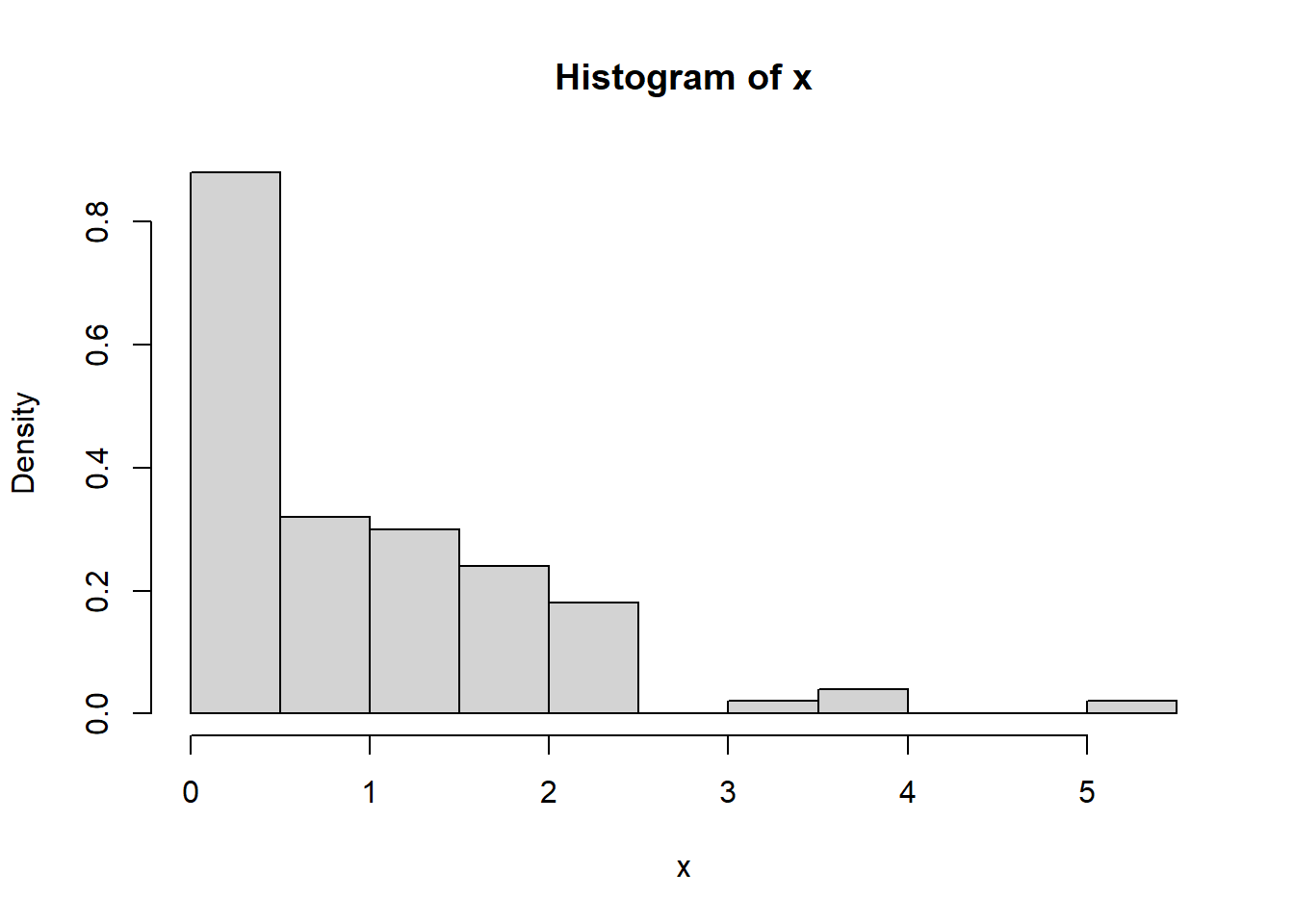# 1 What is R?

R is a free open-source program that is used to do statistics in both academia and industry. There are many online resources for R, for both beginners and experts. For example, Venables, Smith, and the R Core Team

## 1.1 A fancy calculator

R can be used as a fancy calculator

2+2
##  4
sin(3.14)
##  0.001592653
exp(1)
##  2.718282
log(2.71)
##  0.9969486
pi
##  3.141593

Variables can be assigned in the following way

x <- 1+2+3+4+5+6
x*2
##  42

## 1.2 Entering data by hand into R

R is designed to store data as vectors $$x = (x_1, x_2, \ldots, x_n)$$ that is lists of numbers.

y <- c(1,2,3,4,5,6,7,8,9)
y
##  1 2 3 4 5 6 7 8 9

## 1.3 Basic operations with vectors

R has many built in common operations that are useful for statistics:

x <- c(1,2,3,4,5,6,7,8,9,10)
x
##    1  2  3  4  5  6  7  8  9 10
sum(x)
##  55
mean(x)
##  5.5
sd(x)
##  3.02765
var(x)
##  9.166667

R will do certain operations component wise:

 x <- c(1,2,3,4,5)
y <- c(6,7,8,9,10)
z=x+y
z
##   7  9 11 13 15
x*y
##   6 14 24 36 50
sin(z)
##   0.6569866  0.4121185 -0.9999902  0.4201670  0.6502878

It is often necessary to add or delete data from a vector:

x<- c(0.1, 0.2, 0.3, 0.4, 0.5)
x <- x[-5]
x
##  0.1 0.2 0.3 0.4
x <- c(x, 5.5)
x
##  0.1 0.2 0.3 0.4 5.5

## 1.4 Coin-flips, dice roll, normal, and uniform random variables in R

R can be used to simulate coin flips and dice roll. R does not use true randomness, rather it generates coin clips using a deterministic algorithm that simulates true randomness.

x<- rbinom(12,1, 0.5)
x
##   1 0 1 1 0 1 0 0 1 0 1 1
z <- sample(6,12, replace =TRUE)
z
##   3 1 1 5 1 5 5 6 3 6 2 5
x<- rnorm(10, 5, 1)
x
##   5.119908 5.481240 5.276304 4.655133 3.752742 3.945552 4.000917 3.880893
##   6.666759 6.039453
z <- runif(10, min=-1, max=1)
z
##    0.3761206  0.1085798  0.6552083  0.6448328 -0.4148557  0.7324153
##   -0.9345232  0.9837856  0.3563956 -0.8354816

# 2 Histograms in R

x <- rexp(100,1)
hist(x, prob=TRUE)Here $$x$$ is a 100 randomly generated data points from the exponential distribution with rate $$1$$.

# 3 Functions

Suppose we needed to use the quantity $$\sin(x) + \cos(x)$$ over and over again for different values of $$x$$, then it may be useful to define this as a function in the following way:

sincos <- function(x){
z <- sin(x) + cos(x);
z
}
sincos(1)
##  1.381773

Here the function sincos takes an input $$x$$. It is sometimes useful have functions that do not take inputs, but simple perform operations: say roll a fair dice 1o times, and take the sum.

# 4 Basic programming in R

We will introduce basic programming in R with the following exercise and will illustrate how to write a while loop in R.
Exercise 4.1 By running simulations in R, approximate the average number of rolls of a fair dice it takes before you see a 6.

Solution. We make a function numrolls which counts how many times we need to roll a dice until we see a $$6$$. Then we use the replicate command to repeat this function many time, and take the average.

numrolls <- function(){
n=0
x=0
while(x <6){
x <- sample(6,1, replace =TRUE)
n <- n+1
}
n
}

mean(replicate(1000, numrolls()) )
##  5.949

In the next exercise, we illustrate how to write a for loop.

Exercise 4.2 Define a function that tells you whether a positive integer is prime or not.

Solution. Let $$n$$ be an integer. We first recall that $$d$$ is a divisor of $$n$$ if there exists an integer $$c$$ such that $n = cd$. An integer $$n \geq 2$$ is prime if it only divisors are $$1$$ and $$n$$. R has a built in remainder function, which for nonnegative integers $$a,b$$ outputs the remainder in the sense of elementary school, when $$a$$ is divided by $$b$$. Using the remainder function we define the isprime function, and use it spit out the prime numbers up to 500.

25%%5
##  0
26%%5
##  1
isprime <- function(n){
x=1
for (i in 2: (n-1)){
if (x >0) {
x <- n%%i
}
}
if (n==1) {x <-0}
if (n==2) {x <-1}
x
}
isprime(1)
##  0
 isprime(2)
##  1
isprime(101)
##  1
x=2
for(i in 3:500){
if( isprime(i)==1){
x <- c(x, i)}
x
}
x
##     2   3   5   7  11  13  17  19  23  29  31  37  41  43  47  53  59  61  67
##   71  73  79  83  89  97 101 103 107 109 113 127 131 137 139 149 151 157 163
##  167 173 179 181 191 193 197 199 211 223 227 229 233 239 241 251 257 263 269
##  271 277 281 283 293 307 311 313 317 331 337 347 349 353 359 367 373 379 383
##  389 397 401 409 419 421 431 433 439 443 449 457 461 463 467 479 487 491 499

# 5 Summary

We introduced some basics of R, and we gave examples programming basics including defining functions, while loops, for loops, and using the replicate function.